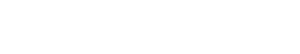# Metrics

Metrics refers to a set of measurements used to evaluate or compare something. Metrics can be used to assess the performance of a system, process, or individual.

There are many different types of metrics that can be used, and the most appropriate metric(s) to use will depend on the specific situation. Some common examples of metrics used in text analytics include accuracy, precision, recall, and F1 score.

It is important to note that the term metrics is sometimes used more broadly to refer to any kind of data or information that can be used to measure something. In this wider sense, metrics can include things like counts, percentages, averages, and other statistical measures.

Thus, when someone outside of the text analytics industry talks about metrics, it is important to ask for clarification on what specific type of data or information they are referring to.

## Types of Metrics

accuracy metric : A metric used to evaluate a model’s classification accuracy. The classification accuracy is the number of correct predictions divided by the total number of predictions.

precision metric: A metric used to evaluate a model’s precision. Precision is the number of true positives divided by the sum of the true positives and false positives.

recall metric: A metric used to evaluate a model’s recall. Recall is the number of true positives divided by the sum of the true positives and false negatives.

F1 score: A metric that combines accuracy and recall into a single measurement. The F1 score is calculated as the harmonic mean of accuracy and recall.

## Importance of Metrics

Metrics are a important part of text analytics because they provide a way to objectively compare different models or methods. Without metrics, it would be difficult to say with confidence which approach is better. However, it is important to remember that no single metric is perfect, and that different metrics will emphasize different aspects of performance. As such, it is usually best to use multiple metrics when evaluating a model.Unlock the power of actionable insights with AI-based natural language processing.